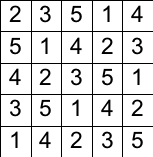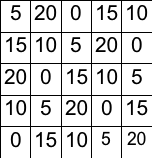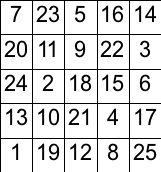When a Belgium canon meets a French mathematician

Let us consider again magic squares, I want to show that it is possible to find solutions for very large squares, using methods completely different from the improved combinatorial method used by CAIA in the preceding post.

In 1703, canon Poignard, from Brussels, found an algorithm that generates solutions for magic squares when N is odd. This method was improved by mathematician Philippe de la Hire, who issued in 1705 a communication to the Académie Royale des Sciences. This method is well known; it was already described in Diderot’s Encyclopedie, entry ‘quarrés magiques’.

For describing this method, I will consider N=5. Let us choose a permutation of the numbers from 1 to N, for instance: 2,3,5,1,4. We write it on the first line of the square. For the second line, we put this permutation shifted by P elements; P is a number less than N such that P and P-1 must be relatively prime numbers with N. This implies that the method cannot be used for even squares: as either P or P-1 is even, N cannot be even. If one chooses P=3, the second line will be 5,1,4,2,3. The process continues, and we get the square just down left.In the same way, we consider a permutation of the integers from 0 to N-1, multiplied by N, for instance: 5,20,0,15,10 which is written on the first line of another square. This new square is completed as the preceding one, with a shift Q, which must also be different from P. With Q=4, we generate the square on the right above.

Both squares have exactly once each number on each line, each column, and each diagonal; moreover, the sums on these lines are the same, since we have the same permutation. Naturally, there is no bijection, since there are only N different numbers for the N2 little squares of each square.

We create the magic square by adding these two squares. It has always all the preceding characteristics, and each value between 1 and N2 is present exactly once.An interesting remark: if opposite sides of the square are considered as next to one another, all the diagonals have also the common value for their sum. We do not generate only magic squares, but a kind of ‘hyper-magic’ square that has 4N constraints, including N diagonals to the right and N diagonals to the left. The new problem is much more constrained than the original one. This explains why there is no hyper-magic square for N=3, where one cannot find a couple P and Q.

This way of generating magic squares is extraordinary: we do not need to write the constraints, the way the solution is created automatically satisfies them. It is no more necessary to find the value of SUM. It is also unnecessary to backtrack.

Without using a computer, I found a magic square for N=11 in 16 minutes. Furthermore, I wrote a 25 lines C program that generates such squares. In only 333 seconds, it generated (but not stored) a solution for N=100,001. It had to find a value for 10,000,200,001 little squares! It is unthinkable to solve such a problem with the methods used by CAIA, and many other constraint satisfaction systems: there are 200,002 constraints, each one has 100,002 unknowns, and the number of possible values for each unknown is greater than ten billion. Studying the formulation of this problem, canon Poignard transformed a constraint satisfaction problem into an algorithm building a large permutation from simpler permutations.

CAIA’s problem solver is very far from such performances. This does not mean that it is an impossible task for an AI system, but that our systems must have a greater capacity for working at the meta level, studying and transforming the formulation of the problem: they must be able to do what canon Poignard did more than 300 years ago.

One thought on “When a Belgium canon meets a French mathematician”

1.Emile Spengler says:

Very nice and detailed good writen article i like it so much thank you for your efforts.